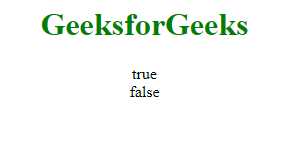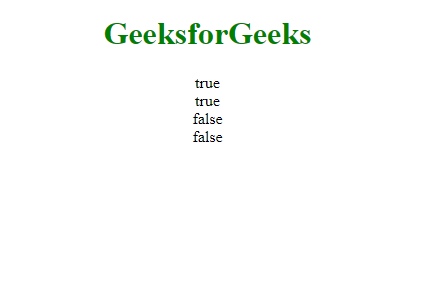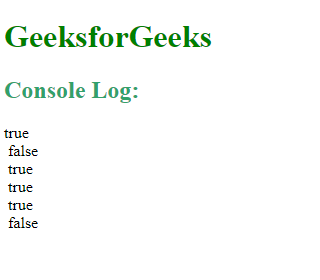# JavaScript Instanceof Operator

Below is the example of the Instanceof Operator.

• Example:

 `  ` `<``html``>  ` ` `  `<``body``>  ` `    ``<``center``>  ` `        ``<``h1` `style``=``"color:green"``>GeeksforGeeks  ` ` `  `        ``<``p` `id``=``"GFG"``>  ` ` `  `        ``<``script``>  ` `            ``var a = ["Geeks", "for", "Geeks"];  ` ` `  `            ``document.getElementById("GFG").innerHTML =  ` `                ``(a instanceof Array) + "<``br``>" +  ` `                ``(a instanceof Number);  ` `        ``  ` `    ``  ` `  ` ` `  `  `

• Output:The instanceof operator in JavaScript is used to check the type of an object at run time. It returns a boolean value if true then it indicates that the object is an instance of a particular class and if false then it is not.

Syntax:

`var gfg = objectName instanceof objectType`

Parameters:
objectName: States the name of Object.

Example-1: objectTypes.

 ` ` `<``html``> ` ` `  `<``body``> ` `    ``<``center``> ` `        ``<``h1` `style``=``"color:green"``>GeeksforGeeks ` ` `  `        ``<``p` `id``=``"GFG"``> ` ` `  `        ``<``script``> ` `            ``var fruits = ["Apple", "Mango", "Banana"]; ` ` `  `            ``document.getElementById("GFG").innerHTML = ` `                ``(fruits instanceof Array) + "<``br``>" + ` `                ``(fruits instanceof Object) + "<``br``>" + ` `                ``(fruits instanceof String) + "<``br``>" + ` `                ``(fruits instanceof Number); ` `        `` ` `    `` ` ` ` ` `  ` `

Output:Example-2: Demonstrating that String and Date objects are also a type of Object (derived from Object).

 ` ` `<``html``> ` ` `  `<``body``> ` `    ``<``h1` `style``=``"color:green"``>GeeksforGeeks ` ` `  `    ``<``p` `id``=``"GFG"``> ` ` `  `    ``<``script``> ` `        ``var myString = new String(); ` `        ``var myDate = new Date(); ` ` `  `        ``console.log(myString instanceof Object); ` `        ``console.log(myString instanceof Date); ` `        ``console.log(myString instanceof String); ` `        ``console.log(myDate instanceof Date); ` `        ``console.log(myDate instanceof Object); ` `        ``console.log(myDate instanceof String); ` `    `` ` ` ` ` `  ` `

OutputSupported Browsers:

• Firefox
• Edge
• Opera
• Apple SafariMy Personal Notes arrow_drop_upCheck out this Author's contributed articles.

If you like GeeksforGeeks and would like to contribute, you can also write an article using contribute.geeksforgeeks.org or mail your article to contribute@geeksforgeeks.org. See your article appearing on the GeeksforGeeks main page and help other Geeks.

Please Improve this article if you find anything incorrect by clicking on the "Improve Article" button below.